# Coax

Click here to go to our main page on microwave transmission lines

Thanks to Daniel, we have images of TE01 and TE11 modes using CST, check it out!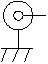Coax symbol

The schematic symbol image for coax shown here is provided in our free schematic symbols download which you can find here.

Coax is unique in that it is the easiest transmission line to analyze, most coax properties can be described with exact closed-form equations. Not like stripline, microstrip or coplanar waveguide...

Go to our download area and get the new Coax.xls spreadsheet. It calculates and plots (over frequency) three types of loss (metal, loss tangent, and dielectric conductivity), and uses a "more exact" metal loss calculation, so it is more accurate (and way more convenient and infinitely cheaper) than EDA software such as Agilent ADS. It just might be our coolest spreadsheet yet! There is also a new multi-dielectric coax spreadsheet in the download area for your enjoyment, thanks to Alex!!

Here are the coax topics covered on this page and other related pages:

Coax overview

Capacitance and inductance per unit length

Characteristic impedance of coax

Cutoff wavelength and frequency

Some industry-standard coax cables

Coax cable vendors

Off-center coax impedance (separate page)

Rectangular (and square) coax (separate page)

Coax power handling (separate page)

Coax loss calculations (separate page)

A more exact calculation of coax loss due to metal (separate page)

The derivation of coax loss due to dielectric loss tangent (separate page)

Loss due to dielectric conduction (separate page)

Why fifty ohms? (separate page)

Multi-dielectric coax (separate page)

### Coax overview

Coaxial cable is the solution to many problems, from wide bandwidth, to low loss and high isolation. Ask your cable company how many miles of it they string just so you can enjoy the next Tyson-Jameson Encounter for 15 seconds. Thanks for the Tyson update, James! Wow, that is a dated reference...

Coax provides the very desirable transverse-electromagnetic (TEM) mode of transmission. The filling factor for coax is unity, and "Keff" is equal to ER. Coax has no lower cutoff frequency (like waveguide does).

A coax transmission line (we prefer the nickname here at Microwaves101 instead of the more academic term "coaxial") consists of two round conductors in which one completely surrounds the other, with the two separated by a continuous solid dielectric (or sometimes by periodic dielectric spacers), as shown below: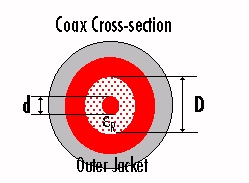### Capacitance and inductance per unit length

These formulas are the exact calculations for capacitance and inductance per length for coax cable. We'll try to stick with the "prime" nomenclature whenever we are talking about quantities that are normalized per unit length.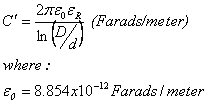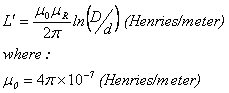Note that the units of D and d don't matter, both calculations only use the ratio D/d. We can simplify them, noting that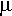R is usually =1 for any dielectric we might be interested in. Then the equations can be expressed in SI units (per meter):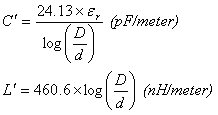or in English units (per foot)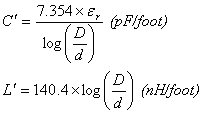### Characteristic impedance

Characteristic impedance is always the square-root of the ratio of inductance per length to capacitance per length: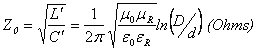This can be simplified to the familiar equation that is shown in almost all coax cable and feedthrough catalogs: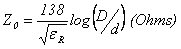Or, perhaps more elegantly,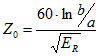Where b and a and the radii of the conductors.  Feel free to replace them with D and d!

### Coax cutoff wavelength and frequency

This material has been moved here.

### Some industry-standard coax cables

This material was moved here.

Author : Unknown Editor